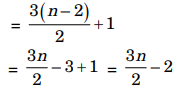# Data Structures and Algorithms - Performance Analysis of Algorithms and Recurrences

>>>>>>>>Performance Analysis of Algorithms and Recurrences

• A

Solves it in linear time using a left to right pass of the array• B

Solves in linear time using a right to left pass• C

Solves it is using divide and conquer in time Θ(n log n)• D

Solves it in time Θ(n2)• Option : B
• Explanation :
The best algorithm is we can solve in linear time using right to left pass.

• A

Takes O(3n) and Ω(2n) time if hashing is permitted• B

Takes O(n3) and Ω(n2.5) time in the key comparison model• C

Takes Θ(n) time and space• D

Takes O(√n) time only if the sum of the 2n• Option : C
• Explanation :
a1, a2 .....................an
b1, b2 .....................bn
In order to find out the largest span, check the sums of
ai +............aj and bi +..............bj at each step.
If a1 + a2 = b1 + b2 go on, check a1 + a2 + a3 and b1 + b2 + b3.
If not, then check a2 + a3 and b2 + b3
Similarly a check is done at each of the n places during traversal. A separate variable has to be kept that contains the maximum span observed hitherto
Hence fastest algorithm computes with (~) (n) time and space.

• A

Heapsort• B

Quicksort• C

Mergesort• D

Radixsort• Option : D
• Explanation :
Radix sort is counting based sorting technical the time complexity is Q(n).

• A

148• B

158• C

184• D

149• Option : A
• Explanation :
From the list of given n numbers [say n is even], Pick up first two elements, compare them
assign Current – min = min of two numbers
Current – max = max of two numbers
From the remaining n – 2 numbers, take pairs wise and follow this process given below.

1. Compare two elements
Assign min = min of two numbers
max = max of two numbers

2. Compare min and current – min
Assign current – min
= min{current– min,min}

3. Compare max and current – max
Assign current – max
= max{current – max, max}

Repeat above procedure for all the remaining pairs of numbers. We can observe that each of pair requires 3 comparisons
1. for finding min and max
2. For updating current – min
3. for updating current – max
But for initial pair we need only one comparison not 3.
∴ Total number of comparisonsHere n =100, so number of comparisons = 148.

• A

O(log n)• B

O(n)• C

O(n log n)• D

O(n2)• Option : B
• Explanation :
Given: n numbers
To find: Tightest upper bound on number of swaps required to sort n numbers using selection sort.
Analysis: In selection sort, in the unsorted part of the array, we find the minimum element and swap it with the value placed at the index where the unsorted array starts.
Hence, for each element to put it in its sorted position, we will do some swaps. In each itenation, when we find the minimum and place it in its sorted position, we do only one swap.
There are n such iterations, since maximum number of positions to sort is n.
Hence, there are n.O(1) swaps
⇒ O(n) swaps.
∴ The solution is (B)